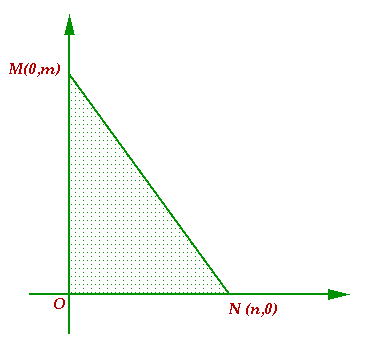#### You may also like### Just Opposite

A and C are the opposite vertices of a square ABCD, and have coordinates (a,b) and (c,d), respectively. What are the coordinates of the vertices B and D? What is the area of the square?A 1 metre cube has one face on the ground and one face against a wall. A 4 metre ladder leans against the wall and just touches the cube. How high is the top of the ladder above the ground?### Beelines

Is there a relationship between the coordinates of the endpoints of a line and the number of grid squares it crosses?

# Hypotenuse Lattice Points

##### Age 14 to 16Challenge Level

 The triangle OMN has vertices on the axes with whole number co-ordinates. Points which have whole number coordinates are called lattice points. How many lattice points are there on the hypotenuse MN?